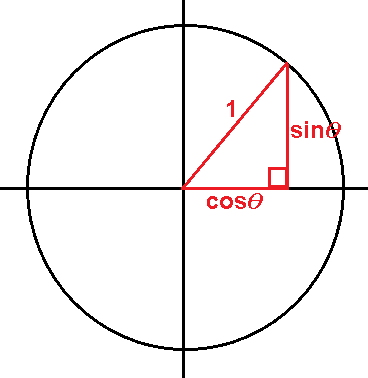# Trigonometric Identities - Day 1 of 2

3 teachers like this lesson
Print Lesson

## Objective

SWBAT simplify expressions and prove trigonometric identities.

#### Big Idea

Identifying what makes a trig expression complex will keep you focused on what needs to be simplified.

## Launch

10 minutes

This lesson is a summation of many of the trigonometric identities that students already know from their work in the previous unit. However, we are going to formalize them as identities and think about the ramifications of that. Begin by writing down sinƟ, cosƟ, and tanƟ on the board and asking students to write expressions in as many different forms as possible.

For sinƟ, we may get the following forms:

1. opp/hyp

2. y/r

3. y

4. 1/cscƟ

My plan is to make an exhaustive list for each of the three trig expressions. Then, we will have a quick conversation about why each statement is true.

## Explore

20 minutes

During the lesson opening, students will likely discuss the reciprocal and quotient properties informally while generating other forms for the Launch activity. To re-start this conversation, I will write down one relationship, such as tanƟ = sinƟ/cosƟ, and explain to the class that this is called an identity. I will ask the class if they know what the word "identity" means in mathematics. If they are not familiar with this term, I will explain that if this is statement is an identity, then the equation is true for any value of Ɵ, with the exception in this case that cosƟ cannot equal zero.

Then, I will have students add these relationships to their Trig Identities worksheet.

To begin our work with Pythagorean identities, I will now show students a diagram like this and see if they can come up with a relationship between sine and cosine:After getting the first Pythagorean identity with sine and cosine, we will work together to derive the other two versions. In my course I emphasize the idea that it is really important that students can derive the other two versions instead of just memorizing them. If students attempt to memorize every identity in this chapter, instead of deriving some, their brain might literally implode.

Now that we have some basic identities, we can start verifying some trig identities. I plan to give students the following expression and ask them to simplify it as much as possible:

sinx/(1+cosx) + cosx/sinx

Then, we will have a quick discussion about what it means to simplify a rational equation so students understand that we want to combine the two fractions and hopefully have no fractions at all. I emphasize that students should try to use the identities that we just learned. This takes time, so I will give my students 5-10 minutes to work on this with their table groups.

## Share

10 minutes

Whenever I am trying to simplify a trig expression or prove a trig identity, I always start by asking myself: what don't I like about this expression? I find that if students take this same approach, figuring out what they don't like about a trig expression, it will give them some guidance as to where to begin (more thoughts about that in this video).

I start our class discussion by asking students what they don't like about the expression sinx/(1+cosx) + cosx/sinx. They will most likely say that they don't like the fractions. Also, since there are two of them, then we want to combine them to be one fraction. Just like with regular numbers, we will need a common denominator to combine fractions, so that will get them started on the right track.

I plan to have a student who got the correct answer share their work with the class. Here is an example of the work. Have the student explain the process and focus on the specific trigonometric identities that they used to simplify. Give students the opportunity to ask questions to the student who presented their work. If a student simplified in a different way, have them share their work too.